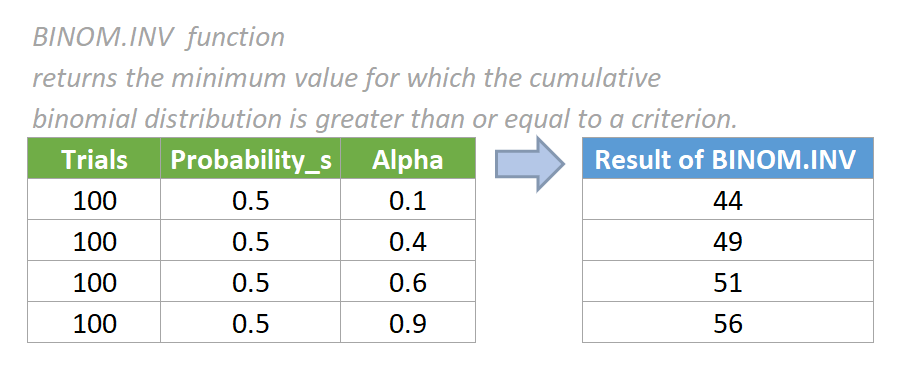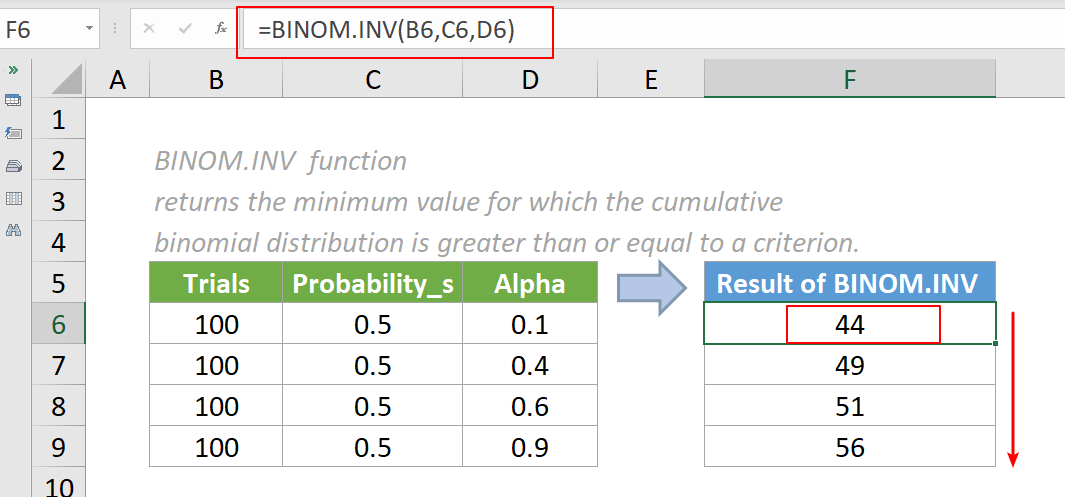Note: The other languages of the website are Google-translated. Back to English

# Excel BINOM.INV Function

## Excel BINOM.INV Function

The BINOM.INV function returns the minimum value for which the cumulative binomial distribution is greater than or equal to a criterion.#### Syntax

=BINOM.INV(trials,probability_s,alpha)

#### Arguments

• Trials (required): The number of independent trials. It must be greater than or equal to 0.
• Probability_s (required): The probability of success in each trial. It must be greater than or equal to 0 and less than or equal to 1.
• Alpha (required): The probability of the Cumulative Binomial Distribution. It must be greater than 0 and less than 1.

#### Return value

The BINOM.INV function returns a numeric value.

#### Function notes

1. If the trials argument is not an integer, the BINOM.INV function will truncate it to an integer.
2. The #VALUE! error value occurs when any of the arguments is non-numeric.
3. The #NUM! error value occurs if:
• the supplied trials argument is < 0
• the supplied probability_s argument is < 0 or > 1
• the supplied alpha argument is < = 0 or > = 1
4. The BINOM.INV function is newly introduced in Excel 2010, so it is not available in earlier versions of Excel.

#### Example

In this case, we want to calculate the smallest value for which the cumulative binomial distribution is greater than or equal to a criterion value based on the data provided in the table below, please do as follows.

1. Please copy the formula below into cell F6, then press the Enter key to get the result.

=BINOM.INV(B6,C6,D6)

2.Select this result cell and drag its autofill handle down to get the rest of the results.Notes:

1. We can also directly input a value in the formula. For example, the formula in cell F6 can be changed to:

=BINOM.INV(100,0.5,0.1)

#### Relative Functions:

• Excel EVEN Function
The EVEN function rounds numbers away from zero to the nearest even integer.

• Excel EXP Function
The EXP function returns the result of the constant e raised to the nth power.

### The Best Office Productivity Tools

#### Kutools for Excel - Helps You To Stand Out From Crowd

Would you like to complete your daily work quickly and perfectly? Kutools for Excel brings 300 powerful advanced features (Combine workbooks, sum by color, split cell contents, convert date, and so on...) and save 80% time for you.

• Designed for 1500 work scenarios, helps you solve 80% Excel problems.
• Reduce thousands of keyboard and mouse clicks every day, relieve your tired eyes and hands.
• Become an Excel expert in 3 minutes. No longer need to remember any painful formulas and VBA codes.
• 30-day unlimited free trial. 60-day money back guarantee. Free upgrade and support for 2 years.#### Office Tab - Enable Tabbed Reading and Editing in Microsoft Office (include Excel)

• One second to switch between dozens of open documents!
• Reduce hundreds of mouse clicks for you every day, say goodbye to mouse hand.
• Increases your productivity by 50% when viewing and editing multiple documents.
• Brings Efficient Tabs to Office (include Excel), Just Like Chrome, Firefox, And New Internet Explorer.